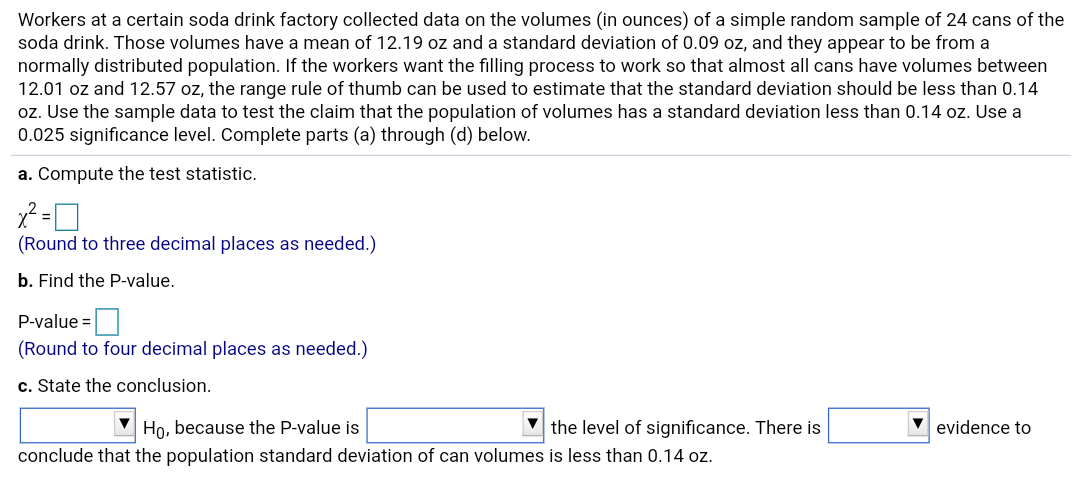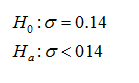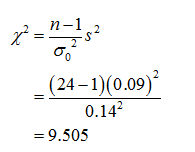# Workers at a certain soda drink factory collected data on the volumes (in ounces) of a simple random sample of 24 cans of thesoda drink. Those volumes have a mean of 12.19 oz and a standard deviation of 0.09 oz, and they appear to be from anormally distributed population. If the workers want the filling process to work so that almost all cans have volumes between12.01 oz and 12.57 oz, the range rule of thumb can be used to estimate that the standard deviation should be less than 0.14oz. Use the sample data to test the claim that the population of volumes has a standard deviation less than 0.14 oz. Use a0.025 significance level. Complete parts (a) through (d) below.a. Compute the test statistic.(Round to three decimal places as needed.)b. Find the P-value.P-value =(Round to four decimal places as needed.)c. State the conclusion.the level of significance. There isHo, because the P-value isevidence toconclude that the population standard deviation of can volumes is less than 0.14 oz.

Question
2 viewshelp_outlineImage TranscriptioncloseWorkers at a certain soda drink factory collected data on the volumes (in ounces) of a simple random sample of 24 cans of the soda drink. Those volumes have a mean of 12.19 oz and a standard deviation of 0.09 oz, and they appear to be from a normally distributed population. If the workers want the filling process to work so that almost all cans have volumes between 12.01 oz and 12.57 oz, the range rule of thumb can be used to estimate that the standard deviation should be less than 0.14 oz. Use the sample data to test the claim that the population of volumes has a standard deviation less than 0.14 oz. Use a 0.025 significance level. Complete parts (a) through (d) below. a. Compute the test statistic. (Round to three decimal places as needed.) b. Find the P-value. P-value = (Round to four decimal places as needed.) c. State the conclusion. the level of significance. There is Ho, because the P-value is evidence to conclude that the population standard deviation of can volumes is less than 0.14 oz. fullscreen
check_circle

Step 1

a).

The sample standard deviation and the sample size are, s = 0.09 and n = 24 respectively. The sample mean is 12.19. The significance level is 0.025. The hypothesis to be tested is,

The null and alternative hypotheses are given below:Step 2

The test is to be performed at 0.025 level of significance.

The value of the test statistic is given and calculated as follows:...

### Want to see the full answer?

See Solution

#### Want to see this answer and more?

Solutions are written by subject experts who are available 24/7. Questions are typically answered within 1 hour.*

See Solution
*Response times may vary by subject and question.
Tagged in

### Statistics